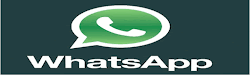Breaking News:

More ...

More ...

More ...

More ...

More ...

More ...

More ...

## Friday, July 5, 2019### Maths Resource material & Teaching Aids for Mathematics subject

Maths Resource material & Teaching Aids for Mathematics subject
I to V  Material for Maths

1. Pebbles Garland (different colours of Pebbels 10 each, total pebbles 100)  10 x10 =100.

2. Loose pebbles – 100.
3. Plastic sticks (length 12 cm each) – 500; big and small rubber bands (1 packet).
4. Unit, tens, hundreds cards (different colours and different sizes each one 20).
5. Flash cards (normal size 1 to 50) – 2 sets.
6. Objects of different things like vegetable, fruit, play things etc. @20 each (small things models).
7. Different shapes of cards @ 20 each (different colours) triangle, square, rectangle, circle etc.
8. 3-D models– cube, cuboids, cone, cylinder, sphere, ring, hemisphere.
9. Cubic blocks. (unit, tens, hundreds) – 4 sets.
10. Model watch.
11. Wall mirror (normal/ big size).
12. Measurements.
a. Containers/ capacity: 1 lt, 2 lt, ½ lt, 200ml, 100ml, 100ml, 50ml.
b. Weight: 1 kg, 2 kg, ½ kg, 200g. 100g, 50g.
c. Length: 1m scale, 30cm scale, 15cm scale, tape.
13. Compass box (big).
14. Dummy currency.
15. Folding paper.
16. Grid sheets.
17. Dot sheets (Isometric sheet).
18. Tangram (5 sets)
19. Dice (different sizes and colour) – 5.
20. Nets of cube, cuboids, (open, closed).
21. 3-D models and objects.
22. Model wood: cube, cuboids, cylinder, cone, sphere etc.
23. Things wood: box, ball, small drum etc.
25. Rubrics cube.
26. Colour papers.
27. Thin end board/ plastic sheet.
28. Cubic boxes – 10.
29. Number chart 1 – 100.
30. Ribbon (containing numbers) 1 to 50/ 1 to 100.
31. Fraction disc.
32. Torch light.
33. Tape/ small scale / big scale.
34. Simple balance.
35. Maths instrument box.
36. Books of Mathematics puzzles, stories and games.
37. Cards with pictures.
38. News magazines.
39. NCERT Mathematics reference books.
40. Reference books related to Mathematics.

VI and VII Material for Maths
1. Population statistics tables – A.P and India (2001 and 2011).
2. Charts: Indo-Arabic and International system (place value charts).
3. Number chart 1 – 100.

6. Panel board.
7. Wall clock.
8. Match sticks/ broom sticks and rubber bands.
9. Card board sheets/ thick sheets/ colour papers,
10. Fraction disc.
11. Model graph sheets, bar graphs, pie graphs charts.
12. Graph sheet, grid chart, grid sheet, isometric chart and sheets.
13. Wall mirror.
14. 3-D shapes – Solids wood – cubes (24), cuboids, sphere, cone, cylinder, prism etc.
15. Angle formation kit.
16. Nets of cube and cuboids etc.
17. Different shapes (triangles, circles, rectangle, square) different sizes and colours – 20 each.
18. Mathematician charts.
19. Compass box (instrument box).
20. Meter scale.
21. Reference books and magazines related to Mathematics.
22. NCERT Mathematics reference books.
23. Fun and fundamentals of Mathematics– Jainath Nilekar and Mangal Nilekar.
24. Pilla Bhashalo Algebra– Namini Subramanya Naidu.
25. Mosco puzzles, 359 Mathematical Recreations– Borris A. Kordemsky.

Material for high school Mathematics
1. Instrument box (compass box) – big size (synthetic material).
2. Plastic coins – two colours - @50 each.
3. Metre scale (wood/ synthetic).

5. Panel board.
6. Metal scale.
7. Wall clock.
8. Bottle caps.
9. 1 cm or 1 inch wooden cubes (100).
10. Mirror.
11. Solid models (wood– cubes (24), cuboids, cylinder, cone, sphere, prism, pyramids).
13. Puzzle books.
14. Tangram.
15. Bank broucher (internet rates – period wise) (banking charts).
16. Square tables (charts).
17. VAT, sales tax (charts).
18. Mathematician charts.
19. Isometric chart.
20. Isometric sheets.
21. Grid paper (chart).
22. Grid paper sheets.
23. 1 – 100 number chart.
24. Graph chart.
25. Tracing papers.
26. Card board.
27. Rubber bands and match sticks/ plastic sticks (2cm length).
28. Seizer, cutter, cutting player.
29. Torch light.
30. Fraction disc.
31. Angle formation kit.
32. Population statics tables (2001 and 2011) India and A.P.
33. Nets of different 3-D shapes (cube, cuboids, cone, pyramid etc.)
34. Reference books and other books related to Mathematics.
35. Magazines related to Mathematics.
36. NCERT Maths reference books.
37. University Press Dictionary of Mathematics– John O E Clark.
38. Short stories about numbers– Rajneesh Kumar.
39. A Premier on number Sequences– Shilesh Sherali.
40. Maths Charmers– Alfred S. Posamentier.
41. Mathematics Maxwells. First Stpes in Number Theory a Primer on divisibility– Shilsh Shirali
42. Themescow Puzzles – 359 Mathematical Recreations– Bories A. Kordemsky.
43. A Biography of the World’s Most Mysterious Number– Alfred S. Posamentier.

Thanks for reading Maths Resource material & Teaching Aids for Mathematics subject